﻿ Passive Earth Pressure - The Sokolovski Theory | Passive Earth Pressure | GEO5 | Online Help

Tree
Settings
Product:
Program:
Language:

## Passive Earth Pressure - The Sokolovski Theory

Passive earth pressure follows from the following formula: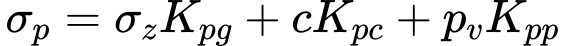where: Kpg - passive earth pressure coefficient for cohesionless soils Kpc - passive earth pressure coefficient due to cohesion Kpp - passive earth pressure coefficient due to  surcharge σz - vertical normal total stress

Individual expressions for determining the magnitude of passive earth pressure and slip surface are introduced in the sequel; the meaning of individual variables is evident from Fig.: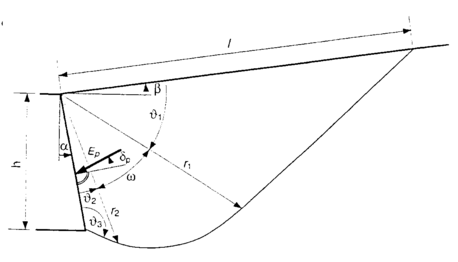Passive eart pressure slip surface after Sokolovski

Angles describing the slip surface:where: φ - angle of internal friction of soil δp - angle of friction between structure and soil β - slope inclination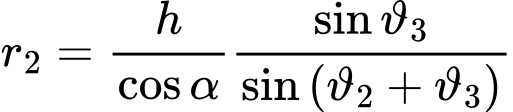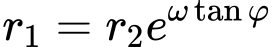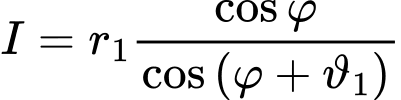Provided that ω < 0 the both straight edges of the zone r1 and r2 numerically overlap and resulting in the plane slip surface developed in the overlapping region. The coefficients of passive earth pressure Kpg, Kpp, Kpc then follow from: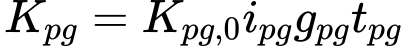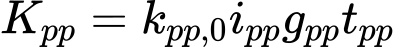where: φ - angle of internal friction of soil δp - angle of friction between structure and soil α - back face inclination of the structureAuxiliary variables: ipg, ipp, ipc, gpg, gpp, gpc, tpg, tpp, tpc

 for: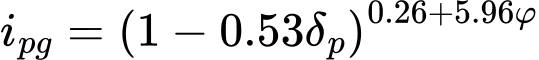,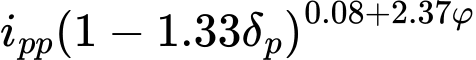,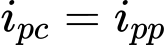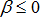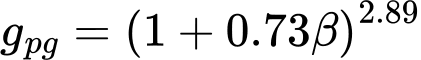,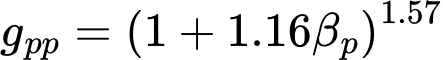,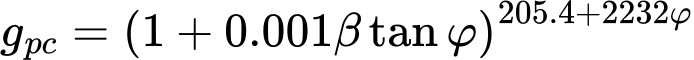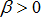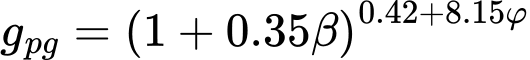,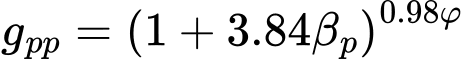,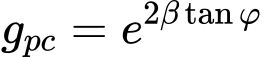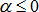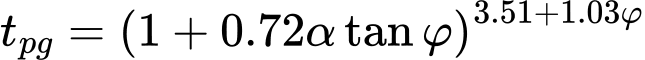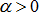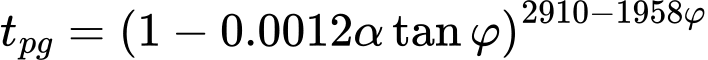where: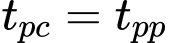For soils with zero value for the angle of internal friction the following expressions are employed to determine the coefficients of passive earth pressure: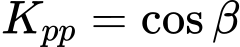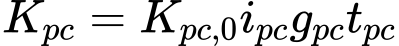where: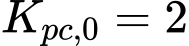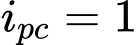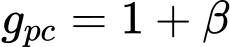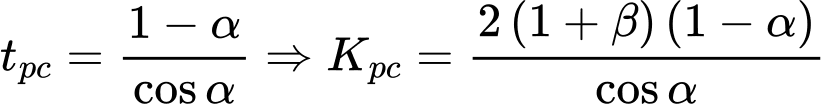Literature:

Sokolovski, V.V., 1960. Statics of Soil Media,Butterworth, London.